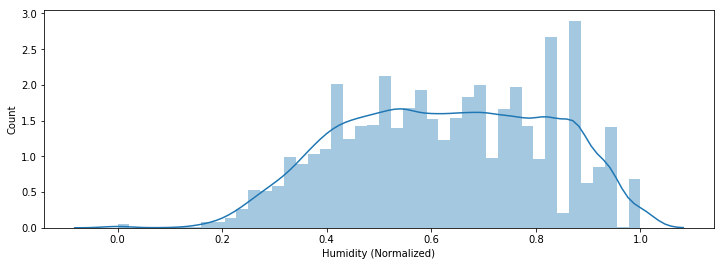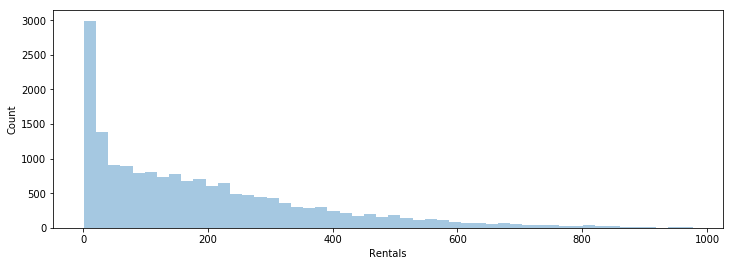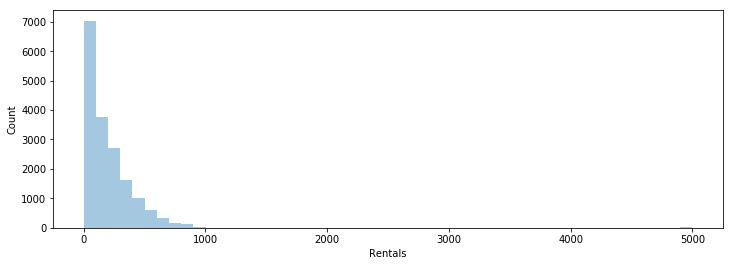In the previous article in this series, we explored the concept of central tendency. The central tedency allows us to grasp the "middle" of the data, but it doesn't tell us anything about the variability of the data. Specifically, how the data is spread out, or the dispersion.

For example, the mean/median/mode of [20, 30, 40, 40, 40, 50, 60] is 40, but the mean/median/mode of [38, 39, 40, 40, 40, 41, 42] is also 40.

The study of dispersion is very important in statistical data. Let's say we are pursuing a job in data science after seeing all the hype arround the field. We are interested in the big money. Well, we can check glassdoor, and find out that the average base pay is ~$120,000. But is that really the pay someone should expect as a junior data scientist? Probably not. The central tendency of the data doesn't tell us the whole story. This is why it is important to also study the dispersion in data. In this exercise, we are going to use the bike sharing dataset from UCI machine learning repository again. For a full description of the dataset, click here. In : import pandas as pd import matplotlib.pyplot as plt import numpy as np import seaborn as sns %matplotlib inline data = pd.read_csv('bike_rental_hour.csv')  ### Measuring Dispersion¶ There are two categories of dispersion measures, and within these two categories there are eight measures I want to talk about. 1) Absolute Measures of Dispersion • Range • Quartile Deviation • Mean/Median Absolute Deviation (MAD) • Variance and Standard Deviation 2) Relative Measures of Dispersion • Coefficient of Range • Coefficient of Quartile Deviation • Coefficient of Mean/Median Deviation • Coefficient of Standard Deviation and Variation I won't go into details with the differences between the two categories. But, I will go in details with each measure. For more details click here. In summary, the absolute measures of dispersion give answers in the same units as the original observations. The relative measures of dispersion are ratios and do not give answers in the same units as the original observations. #### The Range¶ Definition: $$\text{Range} = x_{max} - x_{min}$$ Where$x_{max}$is the maximum value in a given set of observations and$x_{min}$is the minimum value in a given set of observations. The range only tells us about the total spread of data by taking the difference between the minimum and maximum value. The practical uses for the range are very limited in statistics. This measure does have some practical uses in the real world. For example, clothes are often seperated into various size categories. These categories have to fit a certain range of body measurements. The coefficient of range is defined as: $$\text{Coefficient of Range} = \frac{x_{max} - x_{min}} {x_{max} + x_{min}}$$ This equation is the standardized form of$x_{max} - x_{min}$. The coefficient of range is a bit more useful because it allows for comparison across different sets of data. In : print('Range_cnt: {}'.format((data['cnt'].max() - data['cnt'].min()))) print('COR_cnt: {}'.format((data['cnt'].max() - data['cnt'].min())/(data['cnt'].max() + data['cnt'].min()))) print('-----') print('Range_casual: {}'.format((data['casual'].max() - data['casual'].min()))) print('COR_casual: {}'.format((data['casual'].max() - data['casual'].min())/(data['casual'].max() + data['casual'].min())))  Range_cnt: 976 COR_cnt: 0.9979550102249489 ----- Range_casual: 367 COR_casual: 1.0  In the code above, we calculated the range and the coefficient of range for the 'cnt' column and the 'casual' column. The range for the 'cnt' column is 976, and the range for the 'casual' column is 367. This does not imply that the dispersion is greater in the 'cnt' column. The range is an absolute measure specific to the column itself. We have to compare the dispersion using the coefficient of range. In this case, both columns share similar levels of dispersion baesd on the range alone. #### The Quartile Deviation¶ Definition: $$\text{Quartile Deviation} = \frac{Q_{3} - Q_{1}} {2}$$ Where$Q_{3}$is the value at the 75th percentile and$Q_{1}$is the value at the 25th percentile. This measure of dispersion is slightly better than the range if the data set has outliers far away from the center on both sides of the tail. Similar to the range, we are not including the median nor the mean in the calculation. Overall, the practical uses for this measure of dispersion are somewhat limited. The coefficient of quartile deviation is defined as: $$\text{Coefficient of Quartile Deviation} = \frac{Q_{3} - Q_{1}} {Q_{3} + Q_{1}}$$ This equation is the standardized form of the quartile deviation. We can use this coefficient to compare the dispersion of data sets with different variables. #### The Mean/Median Absolute Deviation¶ The arithmetic mean of absolute deviations from the central tendency in a set of observations is defined as the mean deviation, or MAD. The central tendency, or the "middle" of the data can be the mean, the median, or the mode. $$\text{Mean Absolute Deviation} = \frac{\sum\lvert{x} - {x_{center}}\lvert} {n}$$ Where$x$represents a data point,$x_{center}$represents the central tendency,$n$represents the number of rows in a given dataset. This formula represents the average of absolute distances away from the "center" in a given set of data. Unlike the quartile deviation, this measure uses every single data point in a data set in its calculation. The median absolute deviation which is defined as: $$\text{Median Absolute Deviation} = \text{Median} ({\lvert{x_i} - {x_{median}}\lvert})$$ This version of the MAD is more robust than the mean version. Suppose we have a dataset with large values on the tails, the final value will be influenced by the outliers in the calculation of the mean absolute deviation. This is not the case for the median absolute deviation as it will rule out the outliers completely. The coefficient of the mean/median absolute deviation can be calculated by dividing the central tendency of the data: $$\text{Coefficient of Mean/Median Absolute Deviation} = \frac{MAD} {x_{center}}$$ #### The Variance and Standard Deviation¶ The arithmetic mean of squared distances from the average is defined as the variance. $$\text{Variance} = \frac{{\sum({x} - {x_{mean})^2}}} {n}$$ Where$x$represents a data point,$x_{mean}$represents the arithmetic mean,$n\$ represents the number of rows in a given dataset. Similar to the mean absolute deviation, the variance is a useful measurement because it takes in account of every single data point. However, we need to consider that all of the distances are squared, including the outliers. If we have a dataset with outliers far away from average, then these values will be heavily penalized. As a result, the variance will be much higher than the other types of measurement.

The square root of the variance is the standard deviation.

$$\text{Standard Deviation} = \frac{\sqrt{\sum({x} - {x_{mean})^2}}} {n}$$

The standard deviation is the most famous measure of dispersion. The square root portion of this equation lowers the penalty for outliers. However, the influence of outliers will still be higher than the MAD. This measure works very well when the distribution is similar to the gaussian function.

The coefficient of standard deviation can be calculated by dividing the mean of the data:

$$\text{Coefficient of Standard Deviation} = \frac{\text{Standard Deviation}} {x_{mean}}$$

The coefficient of variation is just the percentage form of the coefficient of standard deviation:

$$\text{Coefficient of Variation} = \frac{\text{Standard Deviation}} {x_{mean}} * 100$$

This coefficient is very useful when comparing dispersion of across data sets with different units.

In :
print('CV_cnt: {}%'.format((data['cnt'].std()*100/data['cnt'].mean()).round(1)))
print('CV_hum: {}%'.format((data['hum'].std()*100/data['hum'].mean()).round(1)))

CV_cnt: 95.7%
CV_hum: 30.8%


From the code above, we can say that the variation is higher in the 'cnt' column than the 'hum' column. We are essentially measuring the standard deviation and then representing the result as a percentage ratio of the mean.

The standard deviation is a better representation of the dispersion when the data distribution looks similar to the gaussian function. In large data sets, the histogram often does resemble the normal distribution.

If the data does not resemble the normal distribution, it is actually better to represent the dispersion with the MAD. So I would not rule out the MAD completely.

In :
def mad(data, form='mean'):
if form == 'mean':
return np.mean(np.absolute(data - np.mean(data)))
if form == 'median':
return np.median(np.absolute(data - np.mean(data)))

fig = plt.figure(figsize=(12, 4))
ax = sns.distplot(data['hum'].dropna())
ax.set_ylabel('Count')
ax.set_xlabel('Humidity (Normalized)')
plt.show()

print('standard_deviation_hum: {}'.format((data['hum'].std()).round(3)))standard_deviation_hum: 0.193
mean_absolute_deviation_hum: 0.163
median_absolute_deviation_hum: 0.153


The plot above shows several peaks on the right side of the distribution. Notice how the deviation is lower when we use the median absolute deviation instead of the standard deviation. This is because the mean absolute deviation and standard deviation are penalizing the peaks in this plot.

Let's take a look at an example when the distribution is more skewed:

In :
fig = plt.figure(figsize=(12, 4))
ax = sns.distplot(data['cnt'].dropna(), kde=False)
ax.set_ylabel('Count')
ax.set_xlabel('Rentals')
plt.show()

print('standard_deviation_cnt: {}'.format((data['cnt'].std()).round(3)))standard_deviation_cnt: 181.388
mean_absolute_deviation_cnt: 142.4
median_absolute_deviation_cnt: 131.537


Same thing happened as the previous plot. The mean absolute deviation and standard deviation are penalizing the peaks in this plot. However, this plot is still somehwat similar to the normal distribution.

In :
s1 = pd.Series([5000 for i in range(25)])
test = data['cnt'].append(s1)

fig = plt.figure(figsize=(12, 4))
ax = sns.distplot(test.dropna(), kde=False)
ax.set_ylabel('Count')
ax.set_xlabel('Rentals')
plt.show()

print('standard_deviation_cnt: {}'.format((test.std()).round(3)))standard_deviation_cnt: 257.001
mean_absolute_deviation_cnt: 150.581
median_absolute_deviation_cnt: 134.627


In the plot above, I created a new series named 'test'. Then I added 25 rows to the 'cnt' column with the value of 5,000 each. The default scaling of the histogram picked up a tiny bar at the 5000 mark. The standard deviation jumped from 187 to 257, whereas the two MADs slightly increased.

This paper presented at the British Educational Research Association Annual Conference in 2004 discusses the differences between the MAD and standard deviation in full detail.

#### Summary¶

Determining the best measure of dispersion is something we have to look at in a case by case scenerio. In most cases, the standard deviation will work very well. This is especially true in very large set of data where the normal distribution is well represented. However, it is good practice to always explore the data first and remove outliers if possible.

The files used for this article can be found in my GitHub repository.